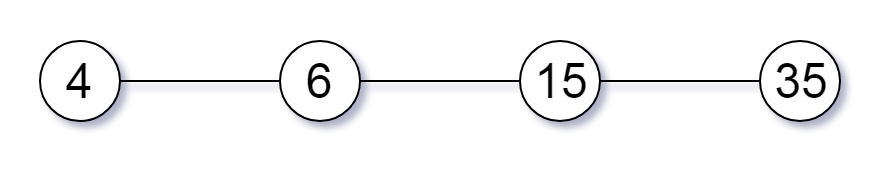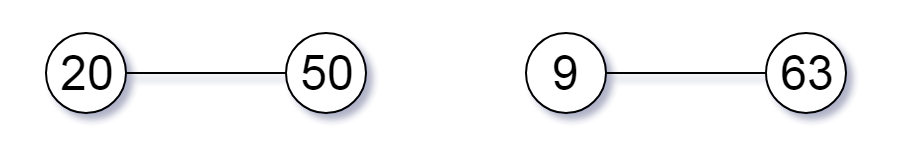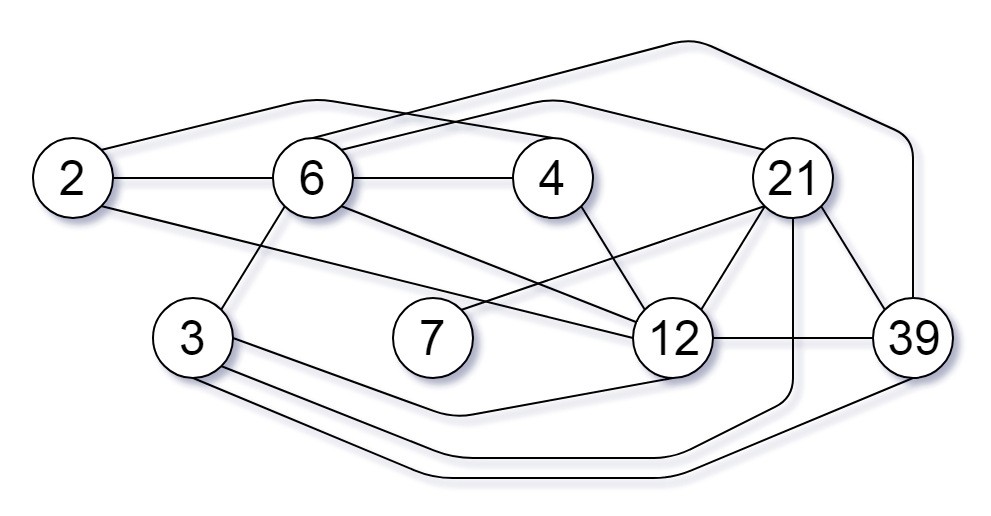# 952. Largest Component Size by Common Factor

Given a non-empty array of unique positive integers `A`, consider the following graph:

• There are `A.length` nodes, labelled `A` to `A[A.length - 1];`
• There is an edge between `A[i]` and `A[j]` if and only if `A[i]` and `A[j]` share a common factor greater than 1.

Return the size of the largest connected component in the graph.

Example 1:

``````Input: [4,6,15,35]
Output: 4
``````Example 2:

``````Input: [20,50,9,63]
Output: 2
``````Example 3:

``````Input: [2,3,6,7,4,12,21,39]
Output: 8
``````Note:

1. `1 <= A.length <= 20000`
2. `1 <= A[i] <= 100000`

``````class Solution {
public:
int largestComponentSize(vector<int>& A) {
int n = 0, mx = 0, res = 0;
unordered_map<int, int> m;
for (int num : A) mx = max(mx, num);
vector<int> root(mx + 1);
for (int i = 1; i <= mx; ++i) root[i] = i;
for (int num : A) {
for (int d = sqrt(num); d >= 2; --d) {
if (num % d == 0) {
root[find(root, num)] = root[find(root, d)];
root[find(root, num)] = root[find(root, num / d)];
}
}
}
for (int num : A) {
res = max(res, ++m[find(root, num)]);
}
return res;
}
int find(vector<int>& root, int x) {
return root[x] == x ? x : (root[x] = find(root, root[x]));
}
};
``````

Github 同步地址:

https://github.com/grandyang/leetcode/issues/952

https://leetcode.com/problems/largest-component-size-by-common-factor/

https://leetcode.com/problems/largest-component-size-by-common-factor/discuss/202053/C%2B%2B-solutions-Easy-to-understandDSU

https://leetcode.com/problems/largest-component-size-by-common-factor/discuss/349437/Java-Simple-O(N*sqrt(W))-Union-Find-Solution

LeetCode All in One 题目讲解汇总(持续更新中…)

 微信打赏Venmo 打赏×

Help us with donation